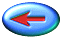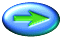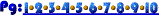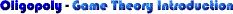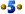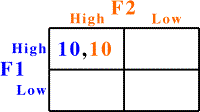Let's consider a simple example of a two firm industry in which the firms can each choose one of two possible strategies. In this case they are the same for the two firms.

Each firm may charge a high price or a low price. Each firm's payoff will depend on what it does and what the other firm does. When we use a payoff matrix like this we are assuming that the firms must either make their decisions at the same time, or that they don't know what the other firm decided until after both firms have chosen.

In this particular case, if each firm charges high prices each will earn payoffs of 10. The first number listed is the payoff to Firm 1 and the second to Firm 2.